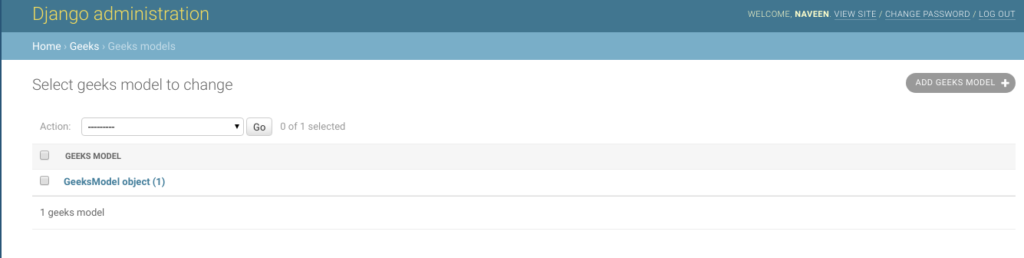# DurationField – Django Models

• Last Updated : 02 Sep, 2021

DurationField is a field for storing periods of time – modeled in Python by timedelta. When used on PostgreSQL, the data type used is an interval and on Oracle the data type is INTERVAL DAY(9) TO SECOND(6). Otherwise, a bigint of microseconds is used. DurationField basically stores a duration, the difference between two dates or times.

Syntax

`field_name = models.DurationField(**options)`

## Django Model DurationField Explanation

Illustration of DurationField using an Example. Consider a project named geeksforgeeks having an app named geeks.

Refer to the following articles to check how to create a project and an app in Django.

Enter the following code into models.py file of geeks app.

## Python3

 `from` `django.db ``import` `models``from` `django.db.models ``import` `Model``# Create your models here.` `class` `GeeksModel(Model):``    ``geeks_field ``=` `models.DurationField()`

Add the geeks app to INSTALLED_APPS

## Python3

 `# Application definition` `INSTALLED_APPS ``=` `[``    ``'django.contrib.admin'``,``    ``'django.contrib.auth'``,``    ``'django.contrib.contenttypes'``,``    ``'django.contrib.sessions'``,``    ``'django.contrib.messages'``,``    ``'django.contrib.staticfiles'``,``    ``'geeks'``,``]`

Now when we run makemigrations command from the terminal,

`Python manage.py makemigrations`

A new folder named migrations would be created in geeks directory with a file named 0001_initial.py

## Python3

 `# Generated by Django 2.2.5 on 2019-09-25 06:00` `from` `django.db ``import` `migrations, models` `class` `Migration(migrations.Migration):` `    ``initial ``=` `True` `    ``dependencies ``=` `[``    ``]` `    ``operations ``=` `[``        ``migrations.CreateModel(``            ``name ``=``'GeeksModel'``,``            ``fields ``=``[``                ``(``'id'``,``                  ``models.AutoField(``                  ``auto_created ``=` `True``,``                  ``primary_key ``=` `True``,``                  ``serialize ``=` `False``,``                  ``verbose_name ``=``'ID'``                ``)),``                ``(``'geeks_field'``, models.DurationField()),``            ``],``        ``),``    ``]`

Now run,

`Python manage.py migrate`

Thus, an geeks_field DurationField is created when you run migrations on the project. It is a field to store datetime.timedelta python object.

## How to use DurationField ?

DurationField is used for storing python datetime.timedelta instance in the database. One can store any type of duration based on time or date in the database. To know more about datetime.timedelta, check out Python | datetime.timedelta() function. Let’s try storing a timedelta instance in model created above.

## Python3

 `# importing the model``# from geeks app``from` `geeks.models ``import` `GeeksModel` `# importing datetime module``import` `datetime` `# creating an instance of``# datetime.timedelta``d ``=` `datetime.timedelta(days ``=``-``1``, seconds ``=` `68400``)` `# creating an instance of``# GeeksModel``geek_object ``=` `GeeksModel.objects.create(geeks_field ``=` `d)``geek_object.save()`

Now let’s check it in admin server. We have created an instance of GeeksModel.## Field Options

Field Options are the arguments given to each field for applying some constraint or imparting a particular characteristic to a particular Field. For example, adding an argument null = True to DurationField will enable it to store empty values for that table in relational database.
Here are the field options and attributes that a DurationField can use.

My Personal Notes arrow_drop_up